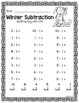# Winter Subtraction Practice: Subtraction Worksheets, Task Cards, Write the RoomSubject
Resource Type
File Type

PDF

(8 MB|67 pages)
Also included in:
1. This is an awesome BUNDLE for addition and subtraction practice. It includes pages for adding and subtracting specific numbers, adding and subtracting using number lines, adding and subtracting using ten frames, task cards, and write the room pages. Each activity includes a fun Winter theme.Also T
\$14.00
\$7.84
Save \$6.16
2. This is an awesome BUNDLE for addition and subtraction practice. It includes pages for adding and subtracting specific numbers, adding and subtracting using number lines, adding and subtracting using ten frames, task cards, and write the room pages. Each activity includes a fun Winter theme.Also T
\$21.00
\$11.76
Save \$9.24
Product Description

This is an awesome product for subtraction practice. It includes worksheets subtracting specific numbers, subtracting using number lines, subtracting using ten frames, task cards, and write the room pages. Each activity includes a fun Winter theme.

Also Try:

Winter Color By Code Bundle: Winter, Christmas, and Valentine's Day

Winter Color By Code Adding and Subtracting Using a Number Line

Fall Subtraction Practice

Fall Color By Code: Adding Using A Number Line

This product includes the following:

Subtracting with Zero

Subtracting with One

Subtracting with Two

Subtracting with Three

Subtracting with Four

Subtracting with Five

Subtracting with Six

Subtracting with Seven

Subtracting with Eight

Subtracting with Nine

Subtracting with Ten

Subtracting Using a 0-10 Number Line (2 pages)

Subtracting Using a 0-20 Number Line (2 pages)

Subtracting Using 1 Ten Frame (2 pages)

Subtracting Using 2 Ten Frames (2 pages)

Subtracting within 10 (2 pages)

Subtracting within 20 (2 pages)

Subtracting Using Color (Subtract within 10)(2 pages)

Subtracting Using Color (Subtract within 20)(2 pages)

Write the Room (Subtract within 10)

Write the Room (Subtract within 20)

Total Pages
67 pages
Included
Teaching Duration
N/A
Report this Resource to TpT
Reported resources will be reviewed by our team. Report this resource to let us know if this resource violates TpT’s content guidelines.\$5.60
List Price:
\$7.00
You Save:
\$1.40